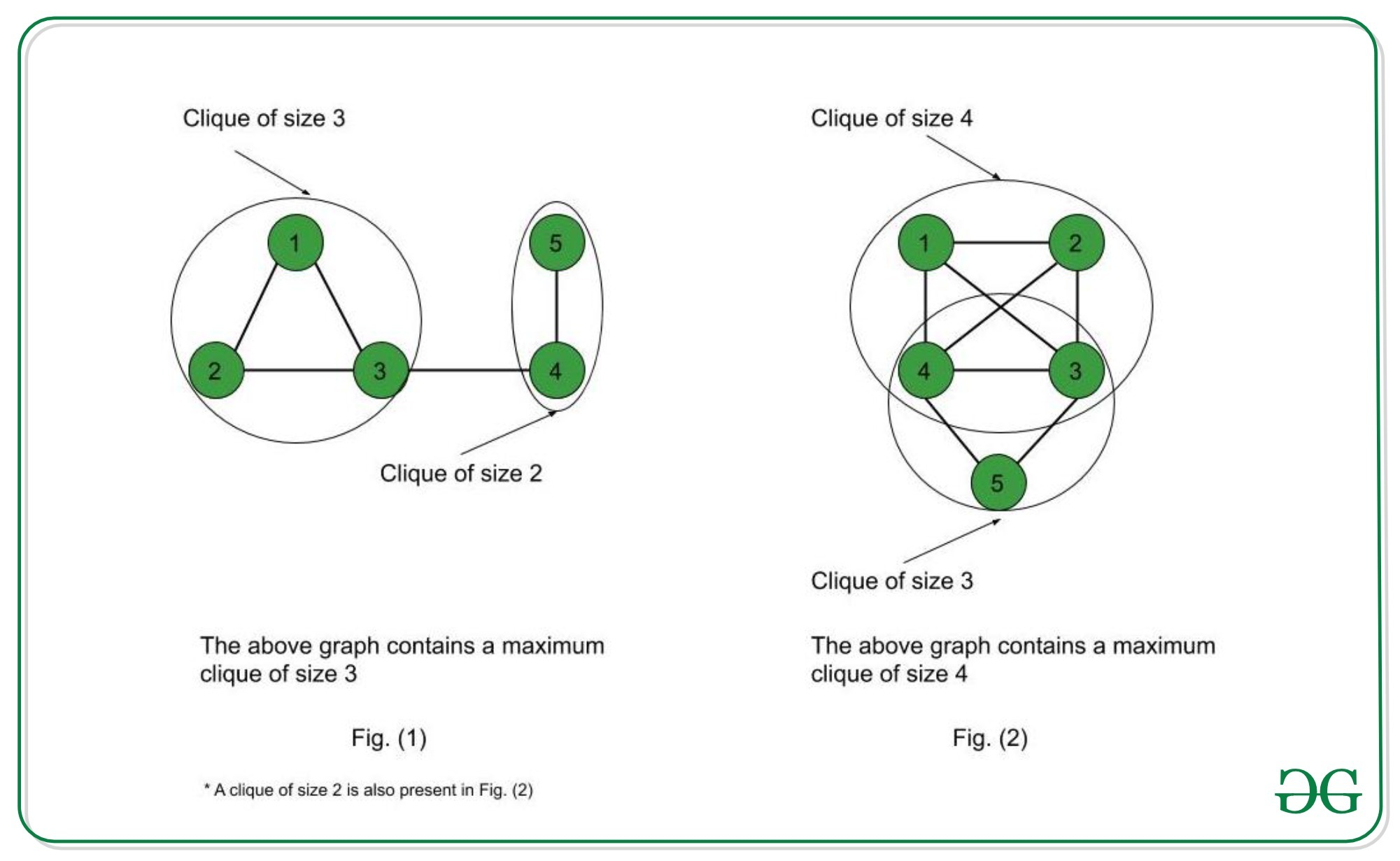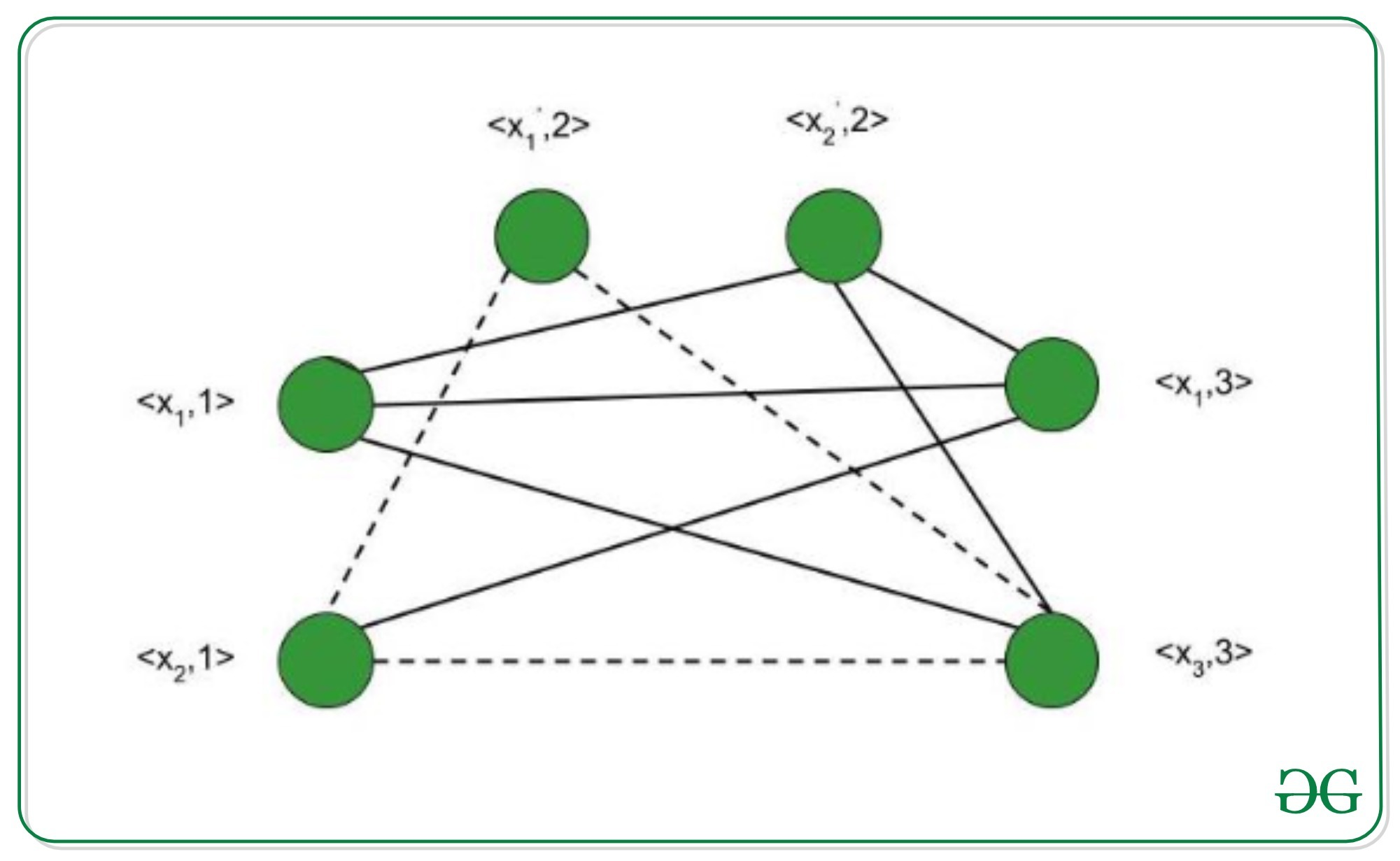# Proof that Clique Decision problem is NP-Complete

Prerequisite: NP-Completeness

A clique is a subgraph of a graph such that all the vertices in this subgraph are connected with each other that is the subgraph is a complete graph. The Maximal Clique Problem is to find the maximum sized clique of a given graph G, that is a complete graph which is a subgraph of G and contains the maximum number of vertices. This is an optimization problem. Correspondingly, the Clique Decision Problem is to find if a clique of size k exists in the given graph or not.To prove that a problem is NP-Complete, we have to show that it belongs to both NP and NP-Hard Classes. (Since NP-Complete problems are NP-Hard problems which also belong to NP)

The Clique Decision Problem belongs to NP – If a problem belongs to the NP class, then it should have polynomial-time verifiability, that is given a certificate, we should be able to verify in polynomial time if it is a solution to the problem.

Proof:

1. Certificate – Let the certificate be a set S consisting of nodes in the clique and S is a subgraph of G.
2. Verification – We have to check if there exists a clique of size k in the graph. Hence, verifying if number of nodes in S equals k, takes O(1) time. Verifying whether each vertex has an out-degree of (k-1) takes O(k2) time. (Since in a complete graph, each vertex is connected to every other vertex through an edge. Hence the total number of edges in a complete graph = kC2 = k*(k-1)/2 ). Therefore, to check if the graph formed by the k nodes in S is complete or not, it takes O(k2) = O(n2) time (since k<=n, where n is number of vertices in G).

Therefore, the Clique Decision Problem has polynomial time verifiability and hence belongs to the NP Class.

The Clique Decision Problem belongs to NP-Hard – A problem L belongs to NP-Hard if every NP problem is reducible to L in polynomial time. Now, let the Clique Decision Problem by C. To prove that C is NP-Hard, we take an already known NP-Hard problem, say S, and reduce it to C for a particular instance. If this reduction can be done in polynomial time, then C is also an NP-Hard problem. The Boolean Satisfiability Problem (S) is an NP-Complete problem as proved by the Cook’s theorem. Therefore, every problem in NP can be reduced to S in polynomial time. Thus, if S is reducible to C in polynomial time, every NP problem can be reduced to C in polynomial time, thereby proving C to be NP-Hard.

Proof that the Boolean Satisfiability problem reduces to the Clique Decision Problem
Let the boolean expression be – F = (x1 v x2) ^ (x1‘ v x2‘) ^ (x1 v x3)  where x1, x2, x3 are the variables, ‘^’ denotes logical ‘and’, ‘v’ denotes logical ‘or’ and x’ denotes the complement of x. Let the expression within each parentheses be a clause. Hence we have three clauses – C1, C2 and C3. Consider the vertices as – <x1, 1>; <x2, 1>; <x1’, 2>; <x2’, 2>; <x1, 3>; <x3, 3> where the second term in each vertex denotes the clause number they belong to. We connect these vertices such that –

1. No two vertices belonging to the same clause are connected.
2. No variable is connected to its complement.Thus, the graph G (V, E) is constructed such that – V = { <a, i> | a belongs to Ci } and E = { ( <a, i>, <b, j> ) | i is not equal to j ; b is not equal to a’ } Consider the subgraph of G with the vertices <x2, 1>; <x1’, 2>; <x3, 3>. It forms a clique of size 3 (Depicted by dotted line in above figure) . Corresponding to this, for the assignment – <x1, x2, x3> = <0, 1, 1>  F evaluates to true. Therefore, if we have k clauses in our satisfiability expression, we get a max clique of size k and for the corresponding assignment of values, the satisfiability expression evaluates to true. Hence, for a particular instance, the satisfiability problem is reduced to the clique decision problem.

Therefore, the Clique Decision Problem is NP-Hard.

Conclusion
The Clique Decision Problem is NP and NP-Hard. Therefore, the Clique decision problem is NP-Complete.

Attention reader! Don’t stop learning now. Get hold of all the important DSA concepts with the DSA Self Paced Course at a student-friendly price and become industry ready.

My Personal Notes arrow_drop_upCheck out this Author's contributed articles.

If you like GeeksforGeeks and would like to contribute, you can also write an article using contribute.geeksforgeeks.org or mail your article to contribute@geeksforgeeks.org. See your article appearing on the GeeksforGeeks main page and help other Geeks.

Please Improve this article if you find anything incorrect by clicking on the "Improve Article" button below.

Article Tags :
Practice Tags :

9

Please write to us at contribute@geeksforgeeks.org to report any issue with the above content.